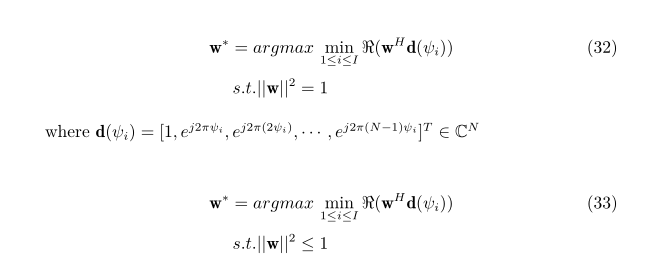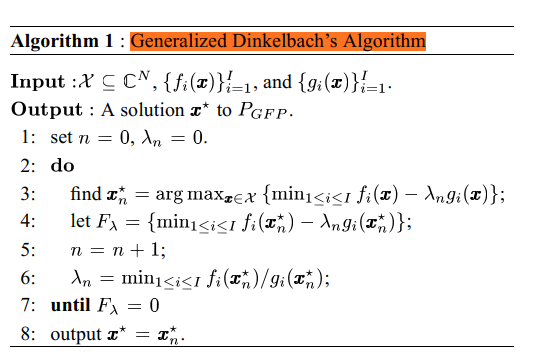# How can I solve this max min problem using CVX?

hello, how can i use cvx to solve this max min problem? this is my code, but something wrong in my programming,can you help me?

clc,clear;
close all;

N=40;
DopTarg=0.4;
Dop=0.4-0.05:0.05:0.4+0.05;
svTarg=exp(1i2pi*(0:N-1)‘DopTarg);
sv=exp(1i
2pi(0:N-1)’*Dop);

% temp=zeros(3,1);
cvx_begin
variable const
% variable temp(3,1)
variable w(N,1) complex
maximize const
subject to
norm(w,2)<=1;
for ii=1:3
temp(ii)=abs(w’*sv(:,ii));
end
const=min(temp);

cvx_end

Have you proven this is a convex optimization problem?

`min(convex)` is not allowed by CVX, because its convexity or concavity can’t be determined as such.

Also note that you need to assign an expression `const`, before using it, as you did in the maximize statement. because you did not, it has the default value of zero at the time of its appearance in the maximize statement; and therefore is the maximizing 0, which is the same as not having an objective at all.

Also, you have changed the norm squared constraint to <= from `, which is needed to make that constraint convex, but is not necessarily equivalent.

I will mark this as a non-convex problem, unless and until you prove otherwise.hello,i’m sorry, it is real not abs in my objective function, and i have solved this problem.hello,can you help me to solve this problem?
I want to use DinkelBech’s algorithm to solve my optimization problem, but something wrong in my programming, can you help me, thank you.

I will only address the CVX error message. I have not looked at anything else.

Maybe you want something like
`norm(sqrtm(Rtotal)*w)`

if Rtotal is (strictly) positive definite, you could alternatively use `chol` instead of `sqrtm`.

I’ll let you figure out whether it is necessary to symmetrize (Hermitianize) or take real part of `Rtotal`, perhaps depending on whether it is theoretically Hermitian.

It seems that you either didn’t read the previously provided link, didn’t understand it, or have not taken it to heart.